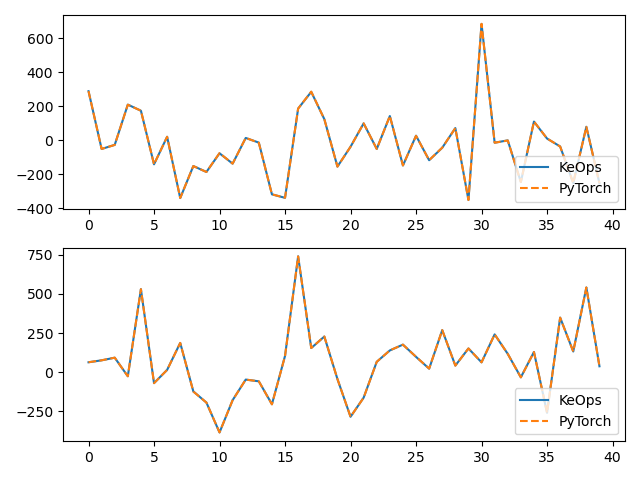# KernelSolve reduction (with LazyTensors)¶

Let’s see how to solve discrete deconvolution problems using the conjugate gradient solver provided by the pykeops.torch.LazyTensor.solve() method of KeOps pykeops.torch.LazyTensor.

## Setup¶

Standard imports:

import time

import torch
from matplotlib import pyplot as plt

from pykeops.torch import LazyTensor as keops
from pykeops.torch import Vi, Vj


Define our dataset:

N = 5000 if torch.cuda.is_available() else 500  # Number of points
D = 2  # Dimension of the ambient space
Dv = 2  # Dimension of the vectors (= number of linear problems to solve)
sigma = .1  # Radius of our RBF kernel

b = torch.rand(N, Dv)
g = torch.Tensor([.5 / sigma ** 2])  # Parameter of the Gaussian RBF kernel
alpha = 0.01  # ridge regularization


Note

This operator uses a conjugate gradient solver and assumes that formula defines a symmetric, positive and definite linear reduction with respect to the alias "b" specified trough the third argument.

Apply our solver on arbitrary point clouds:

print("Solving a Gaussian linear system, with {} points in dimension {}.".format(N, D))
start = time.time()
K_xx = keops.exp(-keops.sum((Vi(x) - Vj(x)) ** 2, dim=2) / (2 * sigma ** 2))
cfun = keops.solve(K_xx, Vi(b), alpha=alpha, call=False)
c = cfun()
end = time.time()
print('Timing (KeOps implementation):', round(end - start, 5), 's')


Out:

Solving a Gaussian linear system, with 5000 points in dimension 2.
Timing (KeOps implementation): 0.30058 s


Compare with a straightforward PyTorch implementation:

start = time.time()
K_xx = alpha * torch.eye(N) + torch.exp(-torch.sum((x[:, None, :] - x[None, :, :]) ** 2, dim=2) / (2 * sigma ** 2))
c_py = torch.solve(b, K_xx)
end = time.time()
print('Timing (PyTorch implementation):', round(end - start, 5), 's')
print("Relative error = ", (torch.norm(c - c_py) / torch.norm(c_py)).item())

# Plot the results next to each other:
for i in range(Dv):
plt.subplot(Dv, 1, i + 1)
plt.plot(c.cpu().detach().numpy()[:40, i], '-', label='KeOps')
plt.plot(c_py.cpu().detach().numpy()[:40, i], '--', label='PyTorch')
plt.legend(loc='lower right')
plt.tight_layout();
plt.show()Out:

Timing (PyTorch implementation): 0.3307 s
Relative error =  0.0003067772777285427
/home/bcharlier/keops/pykeops/examples/pytorch/plot_test_invkernel_torch_helper.py:75: UserWarning: Matplotlib is currently using agg, which is a non-GUI backend, so cannot show the figure.
plt.show()


Compare the derivatives:

print(cfun.callfun)

print("1st order derivative")
e = torch.randn(N, D)
start = time.time()
end = time.time()
print('Timing (KeOps derivative):', round(end - start, 5), 's')
start = time.time()
end = time.time()
print('Timing (PyTorch derivative):', round(end - start, 5), 's')
print("Relative error = ", (torch.norm(u - u_py) / torch.norm(u_py)).item())

# Plot the results next to each other:
for i in range(Dv):
plt.subplot(Dv, 1, i + 1)
plt.plot(u.cpu().detach().numpy()[:40, i], '-', label='KeOps')
plt.plot(u_py.cpu().detach().numpy()[:40, i], '--', label='PyTorch')
plt.legend(loc='lower right')
plt.tight_layout();
plt.show()Out:

<pykeops.torch.operations.KernelSolve object at 0x7fce1c0ada20>
1st order derivative
Timing (KeOps derivative): 0.36614 s
Timing (PyTorch derivative): 0.39162 s
Relative error =  0.0018209443660452962
/home/bcharlier/keops/pykeops/examples/pytorch/plot_test_invkernel_torch_helper.py:102: UserWarning: Matplotlib is currently using agg, which is a non-GUI backend, so cannot show the figure.
plt.show()


Total running time of the script: ( 0 minutes 22.374 seconds)

Gallery generated by Sphinx-Gallery